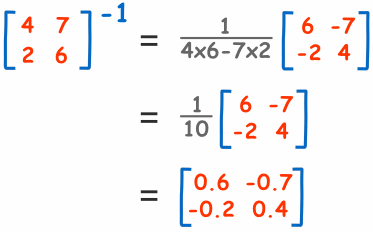#### Asub-routineforthein-situinversionofasquarematrixWhen a programmer deals with mathematics, one of the most common routines added to his/her math library is matrix inversion. The following code implements a very efficient in-situ matrix inversion (meaning that no memory is allocated for another matrix to hold the result; the inverse of the matrix is built gradually in the matrix itself!). The code is based on an algorithm published in Numerical Recipes.

##### Classic VB (vb6) code

The code is written in classic vb (vb6). I am afraid that I do not have the time to convert it to .NET. Have fun:

Public Function TLMatrixInvertGaussJordan(mMatrix1() As Double, gDim As Long) As Long

On Error GoTo Er

'Matrix inversion with Gauss-Jordan elimination and full pivoting.

'mMatrix1 is transformed gradually to its inverse for reasons of memory usage :-)

'if you need to keep the original matrix, copy it to another matrix before inverting!

'Code taken from Numerical Recipes and modified for Visual Basic

'For this version we removed the solution vectors, because we only need the inverse of the matrix

Dim N As Long

Dim nP As Long

Dim i As Long

Dim iCol As Long

Dim iRow As Long

Dim J As Long

Dim K As Long

Dim L As Long

Dim lL As Long

Dim Indxc() As Long

Dim Indxr() As Long

Dim Ipiv() As Long

Dim Big As Double

Dim Dum As Double

Dim piVinv As Double

N = gDim

ReDim Indxc(N) As Long

ReDim Indxr(N) As Long

ReDim Ipiv(N) As Long

For i = 1 To N 'do 22 i=1,n

Big = 0

For J = 1 To N 'do 13 j=1,n

If Ipiv(J) <> 1 Then 'if(ipiv(j).ne.1)then

For K = 1 To N 'do 12 k=1,n

If Ipiv(K) = 0 Then 'if (ipiv(k).eq.0) then

If Abs(mMatrix1(J, K)) >= Big Then ' if (abs(a(j,k)).ge.big)then

Big = Abs(mMatrix1(J, K)) 'Big = Abs(a(J, K))

iRow = J

iCol = K

End If

End If

Next K '12

End If

Next J '13

Ipiv(iCol) = Ipiv(iCol) + 1

If iRow <> iCol Then 'If (iRow.ne.iCol) Then

For L = 1 To N 'do 14 l=1,n

Dum = mMatrix1(iRow, L) '  Dum = a(iRow, L)

mMatrix1(iRow, L) = mMatrix1(iCol, L) 'a(irow,l)=a(icol,l)

mMatrix1(iCol, L) = Dum

Next L '14

'do 15 l=1,m

'Dum = b(iRow, L)

'b(iRow, L) = b(iCol, L)

'b(iCol, L) = Dum

'enddo 15

End If

Indxr(i) = iRow

Indxc(i) = iCol

If Abs(mMatrix1(iRow, iCol)) <= 0.000000000000001 Then 'if (a(icol,icol).eq.0.) pause

Debug.Print "ERROR : TLMatrixInvertGaussJordan : Singular Matrix."

Exit Function

End If

piVinv = 1 / mMatrix1(iCol, iCol) 'pivinv=1./a(icol,icol)

mMatrix1(iCol, iCol) = 1 'a(iCol, iCol) = 1

For L = 1 To N 'do 16 l=1,n

mMatrix1(iCol, L) = mMatrix1(iCol, L) * piVinv 'a(icol,l)=a(icol,l)*pivinv

Next L '16

'do 17 l=1,m

'b(iCol, L) = b(iCol, L) * piVinv

'enddo 17

For lL = 1 To N 'do 21 ll=1,n

If lL <> iCol Then 'if(ll.ne.icol)then

Dum = mMatrix1(lL, iCol) 'dum=a(ll,icol)

mMatrix1(lL, iCol) = 0 'a(ll,icol)=0.

For L = 1 To N 'do 18 l=1,n

mMatrix1(lL, L) = mMatrix1(lL, L) - mMatrix1(iCol, L) * Dum 'a(ll,l)=a(ll,l)-a(icol,l)*dum

Next L ' 18

'do 19 l=1,m

'b(lL, L) = b(lL, L) - b(iCol, L) * Dum

'enddo 19

End If

Next lL '21

Next i '22

For L = N To 1 Step -1 'do 24 l=n,1,-1

If Indxr(L) <> Indxc(L) Then 'if(indxr(l).ne.indxc(l))then

For K = 1 To N 'do 23 k=1,n

Dum = mMatrix1(K, Indxr(L)) 'dum=a(k,indxr(l))

mMatrix1(K, Indxr(L)) = mMatrix1(K, Indxc(L)) 'a(k,indxr(l))=a(k,indxc(l))

mMatrix1(K, Indxc(L)) = Dum 'a(k,indxc(l))=dum

Next K '23

End If

Next L '24

TLMatrixInvertGaussJordan = 1

Exit Function

Er:

Debug.Print "ERROR : TLMatrixInvertGaussJordan : Unspecified error."

End Sub